1. /
2. CBSE
3. /
4. Class 12
5. /
6. Physics
7. /
8. NCERT Solutions class 12...

# NCERT Solutions class 12 physics Nuclei Part 1### myCBSEguide App

Download the app to get CBSE Sample Papers 2023-24, NCERT Solutions (Revised), Most Important Questions, Previous Year Question Bank, Mock Tests, and Detailed Notes.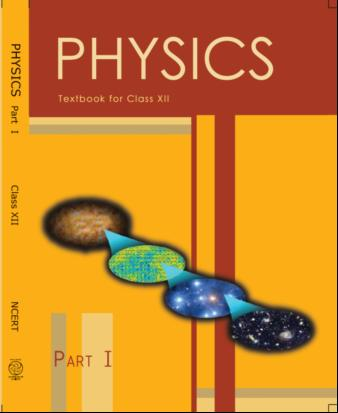## NCERT Class 12 Physics Chapter-wise Solutions

• 1 – Electric Charges and Fields
• 2 – Electrostatic Potential and Capacitance
• 3 – Current Electricity
• 4 – Moving Charges and Magnetism
• 5 – Magnetism and Matter
• 6 – Electromagnetic Induction
• 7 – Alternating Current
• 8 – Electromagnetic Waves
• 9 – Ray Optics and Optical Instruments
• 10 – Wave Optics
• 11 – Dual Nature of Radiation and Matter
• 12 – Atoms
• 13 – Nuclei
• 14 – Semiconductor Electronic: Material, Devices and Simple Circuits
• 15 – Communication Systems

## CHAPTER 13 NUCLEI

• 13.1 Introduction
• 13.2 Atomic Masses and Composition of Nucleus
• 13.3 Size of the Nucleus
• 13.5 Nuclear Force
• 13.7 Nuclear Energy

## NCERT Solutions class 12 physics Nuclei Part 1

1: (a) Two stable isotopes of lithium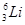and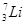have respective abundances of 7.5% and 92.5%. These isotopes have masses 6.01512 u and 7.01600 u, respectively. Find the atomic mass of lithium.

(b) Boron has two stable isotopes,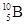and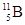. Their respective masses are 10.01294 u and 11.00931 u, and the atomic mass of boron is 10.811 u. Find the abundances ofand.

2: The three stable isotopes of neon: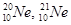and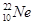have respective abundances of 90.51%, 0.27% and 9.22%. The atomic masses of the three isotopes are 19.99 u, 20.99 u and 21.99 u, respectively. Obtain the average atomic mass of neon.

3: Obtain the binding energy (in MeV) of a nitrogen nucleus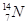, given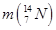=14.00307 u

4:  Obtain the binding energy of the nuclei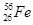and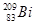in units of MeV from the following data: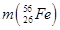= 55.934939 u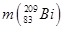= 208.980388 u

5: A given coin has a mass of 3.0 g. Calculate the nuclear energy that would be required to separate all the neutrons and protons from each other. For simplicity assume that the coin is entirely made of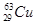atoms (of mass 62.92960 u).

6: Write nuclear reaction equations for

(i) α-decay of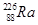(ii) α-decay of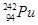(iii)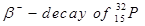(iv)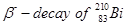(v)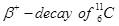(vi)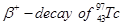(vii) Electron capture of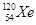7: A radioactive isotope has a half-life of T years. How long will it take the activity to reduce to a) 3.125%, b) 1% of its original value?

8:  The normal activity of living carbon-containing matter is found to be about 15 decays per minute for every gram of carbon. This activity arises from the small proportion of radioactive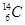present with the stable carbon isotope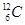. When the organism is dead, its interaction with the atmosphere (which maintains the above equilibrium activity) ceases and its activity begins to drop. From the known half-life (5730 years) of, and the measured activity, the age of the specimen can be approximately estimated. This is the principle ofdating used in archaeology. Suppose a specimen from Mohenjodaro gives an activity of 9 decays per minute per gram of carbon. Estimate the approximate age of the Indus-Valley civilisation.

9:  Obtain the amount of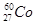necessary to provide a radioactive source of 8.0 mCi strength. The half-life ofis 5.3 years.

10:  The half-life of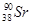is 28 years. What is the disintegration rate of 15 mg of this isotope?

11:  Obtain approximately the ratio of the nuclear radii of the gold isotope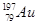and the silver isotope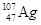.

12:  Find the Q-value and the kinetic energy of the emitted α-particle in the α-decay of (a)and (b)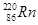. Given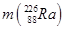= 226.02540 u,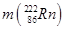= 222.01750 u,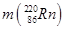= 220.01137 u,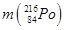= 216.00189 u.

13:  The radionuclide 11C decays according to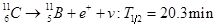The maximum energy of the emitted positron is 0.960 MeV.

Given the mass values: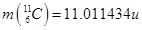and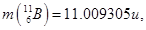calculate Q and compare it with the maximum energy of the positron emitted

14:  The nucleus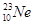decays by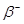emission. Write down the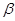decay equation and determine the maximum kinetic energy of the electrons emitted. Given that: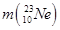= 22.994466 u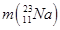= 22.989770 u.

15:  The Q value of a nuclear reaction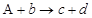is defined by

Q =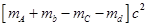where the masses refer to the respective nuclei. Determine from the given data the Q-value of the following reactions and state whether the reactions are exothermic or endothermic.

(i)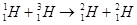(ii)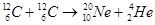Atomic masses are given to be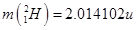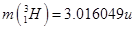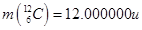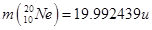## NCERT Solutions for Class 12 Physics

NCERT Solutions Class 12 Physics PDF (Download) Free from myCBSEguide app and myCBSEguide website. Ncert solution class 12 physics includes text book solutions from both part 1 and part 2. NCERT Solutions for CBSE Class 12 Physics have total 20 chapters. 12 Physics NCERT Solutions in PDF for free Download on our website. Ncert physics class 12 solutions PDF and physics ncert class 12 PDF solutions with latest modifications and as per the latest CBSE syllabus are only available in myCBSEguide

To download NCERT Solutions for class 12 Physics, Chemistry, Biology, History, Political Science, Economics, Geography, Computer Science, Home Science, Accountancy, Business Studies and Home Science; do check myCBSEguide app or website. myCBSEguide provides sample papers with solution, test papers for chapter-wise practice, NCERT solutions, NCERT Exemplar solutions, quick revision notes for ready reference, CBSE guess papers and CBSE important question papers. Sample Paper all are made available through the best app for CBSE students and myCBSEguide website.### Test Generator

Create question paper PDF and online tests with your own name & logo in minutes.### myCBSEguide

Question Bank, Mock Tests, Exam Papers, NCERT Solutions, Sample Papers, Notes